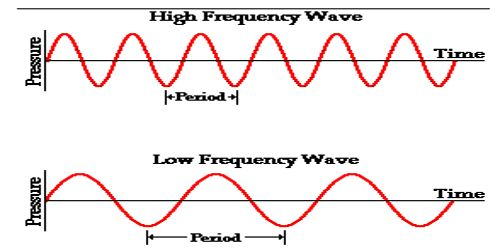# Frequency of Wave

Frequency of Wave

The number of complete oscillations executed by a particle or an object in one second is called the frequency. It is denoted by n or f. So, n = 1/T = N/T

Its unit is cycles per sec (cs-1) or Hertz (Hz).Fig: Frequency of Wave

The frequency, f, of a wave is the number of waves passing a point in a certain time. We normally use a time of one second, so this gives frequency the unit hertz (Hz) since one hertz is equal to one wave per second.

The frequency of a wave describes the number of complete cycles which are completed during a given period of time. As such, the frequency is a rate quantity which describes the rate of oscillations or vibrations or cycles or waves on a per second basis. A common unit of frequency is the Hertz, abbreviated as Hz. A Hertz indicates the number of complete cycles per second.